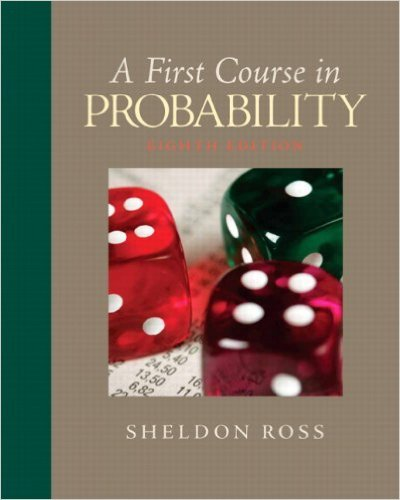×
Get Full Access to First Course In Probability - 8 Edition - Chapter 8 - Problem 8.16
Get Full Access to First Course In Probability - 8 Edition - Chapter 8 - Problem 8.16

×

# A.J. has 20 jobs that she must do in sequence, withtheISBN: 9780136033134 163

## Solution for problem 8.16 Chapter 8

First Course in Probability | 8th Edition

• Textbook Solutions
• 2901 Step-by-step solutions solved by professors and subject experts
• Get 24/7 help from StudySoup virtual teaching assistantsFirst Course in Probability | 8th Edition

4 5 1 357 Reviews
29
3
Problem 8.16

A.J. has 20 jobs that she must do in sequence, withthe times required to do each of these jobs beingindependent random variables with mean 50 minutesand standard deviation 10 minutes. M.J. has20 jobs that he must do in sequence, with the timesrequired to do each of these jobs being independentrandom variables with mean 52 minutes andstandard deviation 15 minutes.(a) Find the probability that A.J. finishes in lessthan 900 minutes.(b) Find the probability that M.J. finishes in lessthan 900 minutes.(c) Find the probability that A.J. finishesbefore M.J.

Step-by-Step Solution:
Step 1 of 3

Review of Notes 4: i = 1, 2 ….. n j = 1, 2 ….r i ̅ ∑ ∑ ̅ ∑ ∑ ∑ ∑ ( ̅) => Overall Variation (SST total sum of squares) ∑ ∑ ( ) = {( ̅ ) ̅ ̅ } = ∑ ∑ ( ̅ ) ∑ ∑ (̅ ̅) ∑ ∑ ( ̅ )(̅ ̅) ∑ ∑ ( ̅ ) Error sum of ∑ ∑ (̅ ̅) mean sum of squares (SSE) squares (SSM Look at ∑ ∑ ( ̅ )(̅ ̅) ∑ ( ̅ ̅)∑ ( ̅ ) ∑ ̅ ∑ ∑ ( ̅) ( ) ∑ (̅ ̅) ( )

Step 2 of 3

Step 3 of 3

##### ISBN: 9780136033134

This full solution covers the following key subjects: minutes, jobs, Probability, Find, finishes. This expansive textbook survival guide covers 10 chapters, and 495 solutions. The full step-by-step solution to problem: 8.16 from chapter: 8 was answered by , our top Statistics solution expert on 11/23/17, 05:06AM. Since the solution to 8.16 from 8 chapter was answered, more than 482 students have viewed the full step-by-step answer. This textbook survival guide was created for the textbook: First Course in Probability, edition: 8. First Course in Probability was written by and is associated to the ISBN: 9780136033134. The answer to “A.J. has 20 jobs that she must do in sequence, withthe times required to do each of these jobs beingindependent random variables with mean 50 minutesand standard deviation 10 minutes. M.J. has20 jobs that he must do in sequence, with the timesrequired to do each of these jobs being independentrandom variables with mean 52 minutes andstandard deviation 15 minutes.(a) Find the probability that A.J. finishes in lessthan 900 minutes.(b) Find the probability that M.J. finishes in lessthan 900 minutes.(c) Find the probability that A.J. finishesbefore M.J.” is broken down into a number of easy to follow steps, and 86 words.

Unlock Textbook Solution